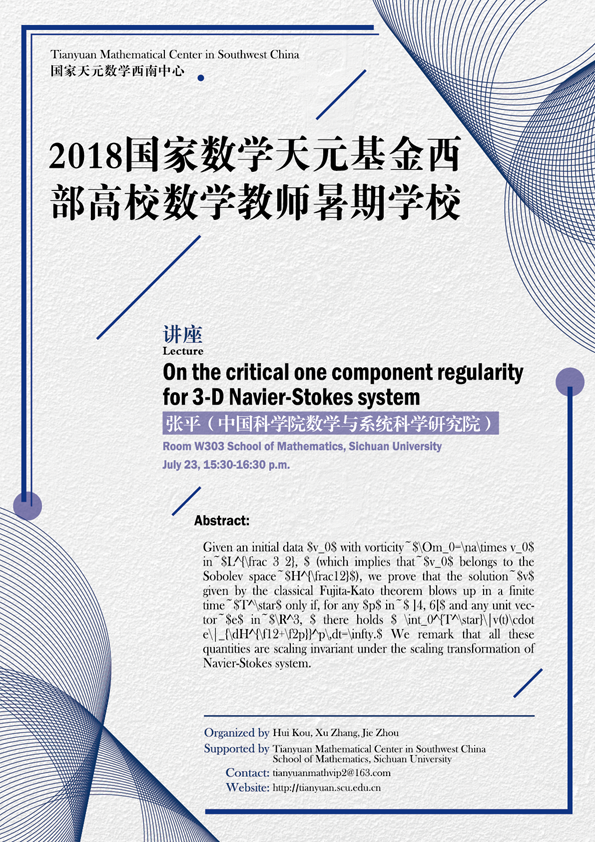On the critical one component regularity for 3-D Navier-Stokes system

#### W303  School of Mathematics, Sichuan University#### ABSTRACT

Given an initial data $v_0$ with vorticity~$\Om_0=\na\times v_0$ in~$L^{\frac 3 2},$ (which implies that~$v_0$ belongs to the Sobolev space~$H^{\frac12}$), we prove that the solution~$v$ given by the classical Fujita-Kato theorem blows up in a finite time~$T^\star$ only if, for any $p$ in~$]4, 6[$ and any unit vector~$e$ in~$\R^3,$ there holds $\int_0^{T^\star}\|v(t)\cdot e\|_{\dH^{\f12+\f2p}}^p\,dt=\infty.$ We remark that all these quantities are scaling invariant under the scaling transformation of Navier-Stokes system.

#### ORGANIZERS

Hui Kou (Sichuan University)

Xu Zhang (Sichuan University)

Jie Zhou (Sichuan University)

#### SUPPORTED BY

Tianyuan Mathematical Center in Southwest China

School of Mathematics, Sichuan University

#### VIDEO

• On the critical one component regularity for 3-D Navier-Stokes system
• 15:30 - 16:30, 2018-07-23
• 张平（中国科学院数学与系统科学研究院）